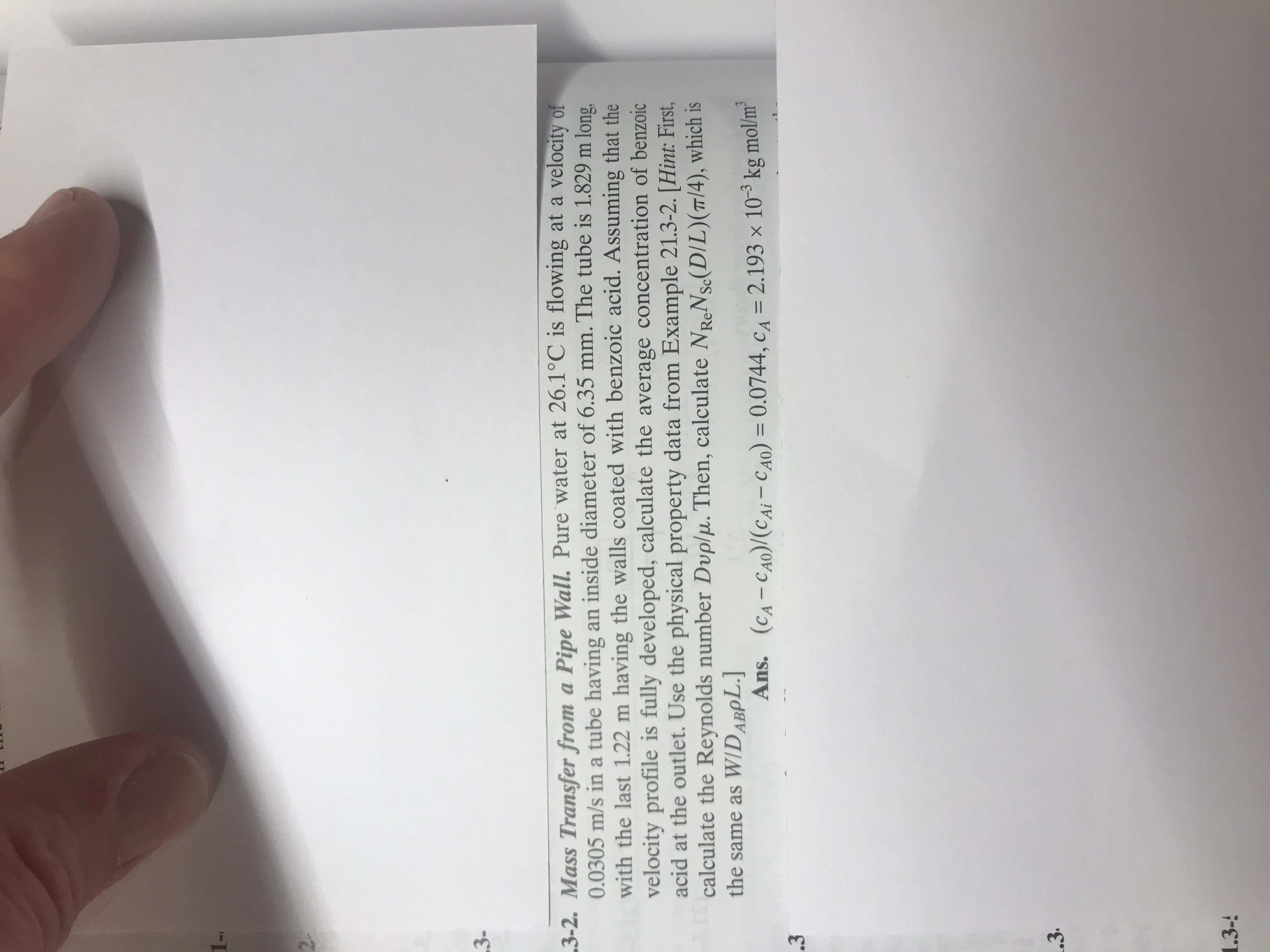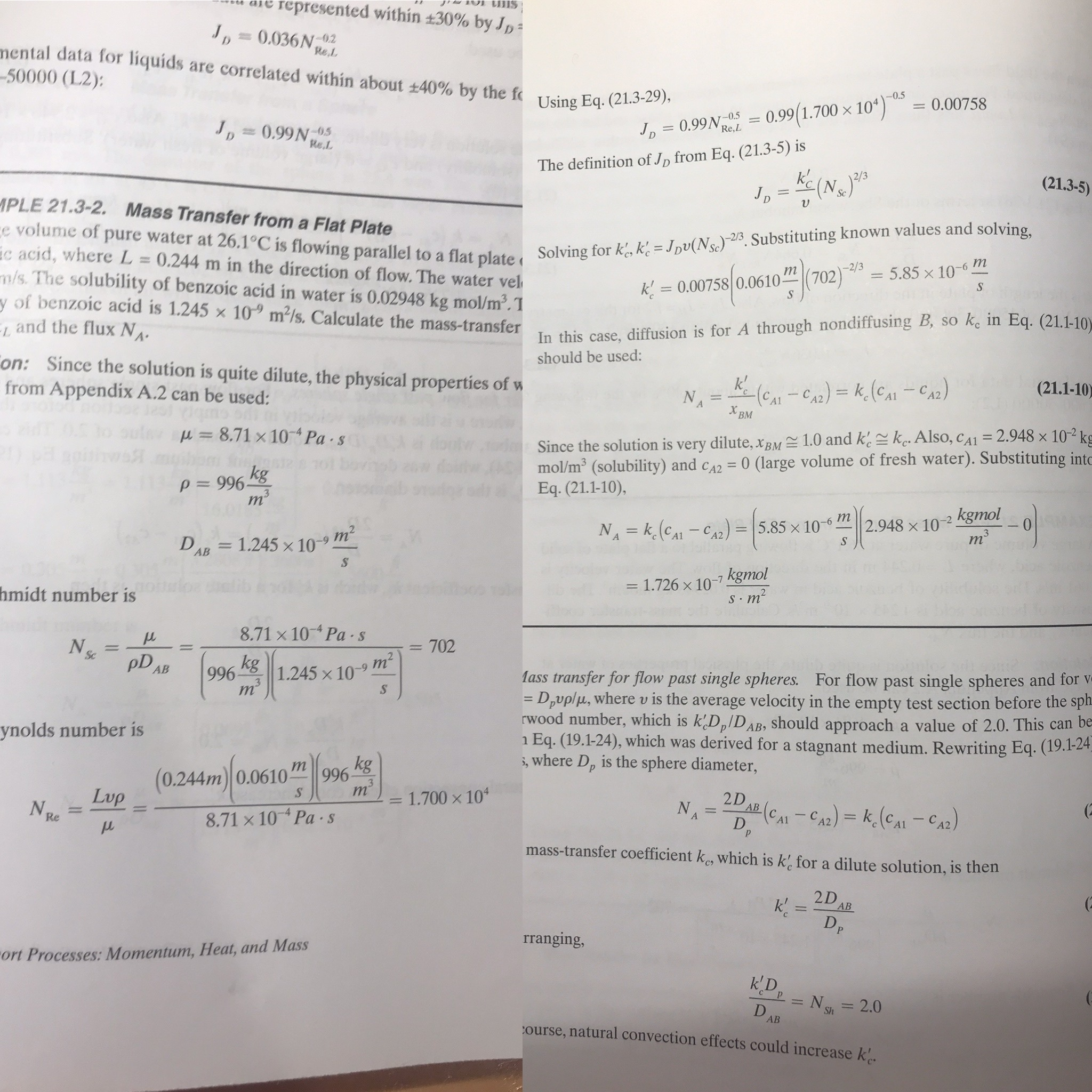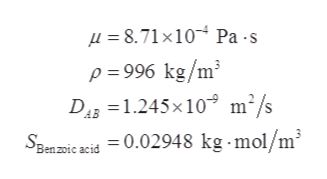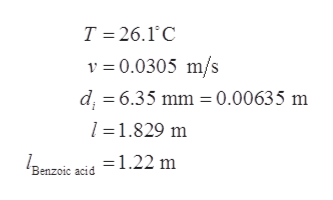# 1M3-3-2. Mass Transfer from a Pipe Wall. Pure water at 26.1°C is flowing at a velocity of0.0305 m/s ina tube having an inside diameter of 6.35 mm. The tube is 1.829 m longwith the last 1.22 m having the walls coated with benzoic acid. Assuming that thevelocity profile is fully developed, calculate the average concentration of benzoicacid at the outlet. Use the physical property data from Example 21.3-2. [Hint: First,calculate the Reynolds number Duplu. Then, calculate NReNse(D/L) (T/4), which isthe same as WIDABPL.Ans.(CA-CAO)/(CAi-CAO) = 0.0744, cA 2.193 x 103 kg mol/m.3.31.3- represented within +30 % by JpSmJD0.036N2mental data for liquids are correlated within about +40% by the f-50000 (L2):Re,L-0.5Using Eq. (21.3-29),0.007580.99(1.700 x 10Jp 0.99N05JD0.99N0.5Re,LRe.LThe definition of Jp from Eq. (21.3-5) iske(Ne(21.3-5)MPLE 21.3-2.Mass Transfer from a Flat Platee volume of pure water at 26.1°C is flowing parallel to a flat plate Solving for ke k= Jpv(Nc)2. Substituting known values and solving,ic acid, where L 0.244 m in the direction of flow. The water velm/s. The solubility of benzoic acid in water is 0.02948 kg mol/m2.5.85 x 10-6 mS-2/3k0.00758 0.0610Sy of benzoic acid is 1.245 x 10 m2/s. Calculate the mass-transfer In this case, diffusion is for A through nondiffusing B, so k, in Eq. (21.1-10)and the flux NAon: Since the solution is quite dilute, the physical properties of wfrom Appendix A.2 can be used:should be used:(e-CA)= k (eA-C2)Х вм(21.1-10)ACubp8.71 x 10 Pa s1.0 and keke Also, cA1 = 2.948 x 10-2 ks0 (large volume of fresh water). Substituting intoSince the solution is very dilute, XBMmol/m3 (solubility) and CA2Eq. (21.1-10),P 996 kgm3kgmolт2.948 x 100NA k.(CAI-CA2) = |5.85 x 10-6m3m2DAR 1.245 x 10S-91.726 x 10-7 kgmolS.m2hmidt number is8.71 x 10 Pa sNo702Sckg996m3pDd mtem2AB1.245 x 10-9Mass transfer for flow past single spheres. For flow past single spheres and for v= D,uplu, where v is the average velocity in the empty test section before the sphrwood number, which is k'D,/DAB, should approach a value of 2.0. This can beEq. (19.1-24), which was derived for a stagnant medium. Rewriting Eq. (19.-24.,where Dp is the sphere diameter,ynolds number iskg(0.244m 0.0610Lup996m21.700 x 102DABNACA-CA)= k(CA- CA2)8.71x 10 Pa -sRemass-transfer coefficient ke, which is k' for a dilute solution, is then2DАВDPIrranging,ort Processes: Momentum, Heat, and MassP N 2.0DАВShourse, natural convection effects could increase k..

Question
273 viewshelp_outlineImage Transcriptionclose1M 3- 3-2. Mass Transfer from a Pipe Wall. Pure water at 26.1°C is flowing at a velocity of 0.0305 m/s ina tube having an inside diameter of 6.35 mm. The tube is 1.829 m long with the last 1.22 m having the walls coated with benzoic acid. Assuming that the velocity profile is fully developed, calculate the average concentration of benzoic acid at the outlet. Use the physical property data from Example 21.3-2. [Hint: First, calculate the Reynolds number Duplu. Then, calculate NReNse(D/L) (T/4), which is the same as WIDABPL. Ans. (CA-CAO)/(CAi-CAO) = 0.0744, cA 2.193 x 103 kg mol/m .3 .3 1.3- fullscreenhelp_outlineImage Transcriptioncloserepresented within +30 % by Jp Sm JD0.036N2 mental data for liquids are correlated within about +40% by the f -50000 (L2): Re,L -0.5 Using Eq. (21.3-29), 0.00758 0.99(1.700 x 10 Jp 0.99N05 JD0.99N0.5 Re,L Re.L The definition of Jp from Eq. (21.3-5) is ke(Ne (21.3-5) MPLE 21.3-2. Mass Transfer from a Flat Plate e volume of pure water at 26.1°C is flowing parallel to a flat plate Solving for ke k= Jpv(Nc)2. Substituting known values and solving, ic acid, where L 0.244 m in the direction of flow. The water vel m/s. The solubility of benzoic acid in water is 0.02948 kg mol/m2. 5.85 x 10-6 m S -2/3 k0.00758 0.0610 S y of benzoic acid is 1.245 x 10 m2/s. Calculate the mass-transfer In this case, diffusion is for A through nondiffusing B, so k, in Eq. (21.1-10) and the flux NA on: Since the solution is quite dilute, the physical properties of w from Appendix A.2 can be used: should be used: (e-CA)= k (eA-C2) Х вм (21.1-10) A Cubp 8.71 x 10 Pa s 1.0 and keke Also, cA1 = 2.948 x 10-2 ks 0 (large volume of fresh water). Substituting into Since the solution is very dilute, XBM mol/m3 (solubility) and CA2 Eq. (21.1-10), P 996 kg m3 kgmol т 2.948 x 10 0 NA k.(CAI-CA2) = |5.85 x 10-6 m3 m2 DAR 1.245 x 10 S -9 1.726 x 10-7 kgmol S.m2 hmidt number is 8.71 x 10 Pa s No 702 Sc kg 996 m3 pD d mte m2 AB 1.245 x 10-9 Mass transfer for flow past single spheres. For flow past single spheres and for v = D,uplu, where v is the average velocity in the empty test section before the sph rwood number, which is k'D,/DAB, should approach a value of 2.0. This can be Eq. (19.1-24), which was derived for a stagnant medium. Rewriting Eq. (19.-24. ,where Dp is the sphere diameter, ynolds number is kg (0.244m 0.0610 Lup 996 m2 1.700 x 10 2D AB NA CA-CA)= k(CA- CA2) 8.71x 10 Pa -s Re mass-transfer coefficient ke, which is k' for a dilute solution, is then 2D АВ DP Irranging, ort Processes: Momentum, Heat, and Mass P N 2.0 D АВ Sh ourse, natural convection effects could increase k.. fullscreen
check_circle

star
star
star
star
star
1 Rating
Step 1

Physical property data to be used from example 21.3-2 are:help_outlineImage Transcriptionclose8.71x10 Pa -s p=996 kg/m3 DA 1.245x10 m2/s = 0.02948 kg mol/m3 SBenz0ic acid 0.02948 kg mol/m2 fullscreen
Step 2

Given data to be used further:help_outlineImage TranscriptioncloseT 26.1 C v 0.0305 m/s d 6.35 mm 0.0063 5 m -1.829 m =1.22 m Benzoic acid fullscreen
Step 3

Calculate the value of S...

### Want to see the full answer?

See Solution

#### Want to see this answer and more?

Solutions are written by subject experts who are available 24/7. Questions are typically answered within 1 hour.*

See Solution
*Response times may vary by subject and question.
Tagged in

### Mass Transfer